# Calculating the Single Amount (PV)

If we know the future value (FV), the number of time periods of compound interest (n), and the interest rate (i), we can use future value factors to calculate the unknown amount that was originally deposited (the "present value," or PV). Calculations #13 through #16 illustrate how to determine the present value (PV).

(Note: The single amount can also be calculated by using present value factors. This is discussed in the AccountingCoach topic Present Value of a Single Amount.)

### Calculation #13

Joan wishes to make one deposit today into an individual retirement account (IRA) that is guaranteed to earn 6% per year compounded annually. She wants the amount deposited to grow to \$10,000 at the end of 12 years. How much will she need to deposit today?

The following timeline plots the variables that are known and unknown: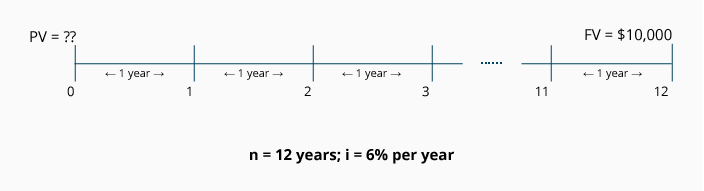Calculation using the FV of 1 Table: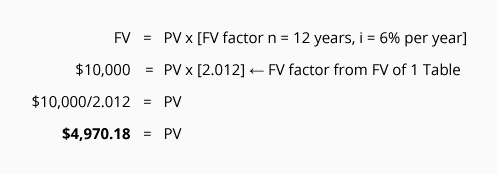In order to have a future value of \$10,000 in 12 years, Joan must deposit \$4,970.18 today in her IRA.

### Calculation #14

What amount will you need to invest today in order to have \$15,000 at the end of 10 years? Assume your amount will earn 10% per year compounded semiannually.

The following timeline plots the variables that are known and unknown: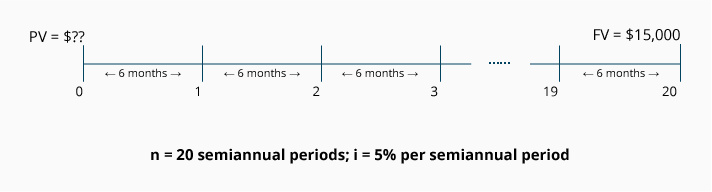Because the interest is compounded semiannually, we convert the 10 annual time periods to 20 semiannual time periods. Similarly, the interest rate is converted from 10% per year to 5% per semiannual period.

Calculation using the FV of 1 Table: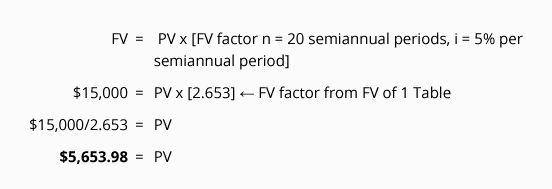You need to invest \$5,653.98 today in order to have it grow to \$15,000 in 20 six-month periods with interest at 10% per year compounded semiannually.

### Calculation #15

What amount today will grow to \$30,000 at the end of 7 years if the amount earns 8% per year compounded quarterly?

The following timeline plots the variables that are known and unknown: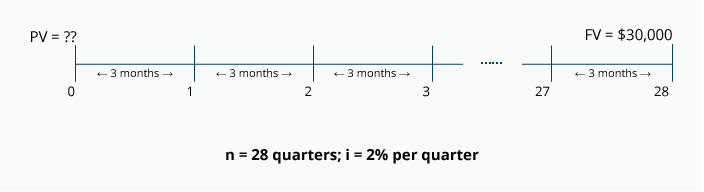Because the interest is compounded quarterly, we convert the 7 one-year time periods to 28 quarters. Similarly, the interest rate is converted from 8% per year to 2% per quarter. In other words, n = 28 quarters, and i = 2% per quarter.

Calculation using the FV of 1 Table: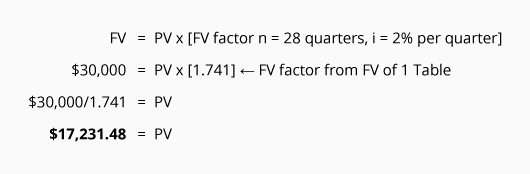A single deposit of \$17,231.48 will grow to \$30,000 if it remains invested at 8% per year compounded quarterly for 7 years.

### Calculation #16

The number of visitors to Bill's website is increasing at an annual rate of 36% compounded monthly. By the end of one year Bill expects the number of visitors to his site to reach 50,000 per day. What is the present number of visitors per day?

The following timeline plots the variables that are known and unknown: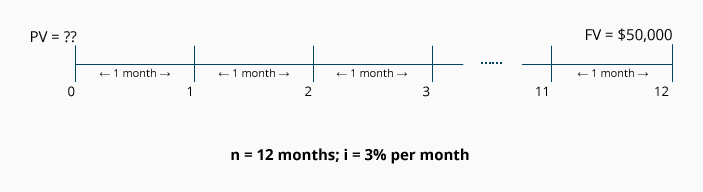Because the rate is compounded monthly, we convert the one-year time period to 12 monthly time periods. Similarly, the rate is converted from 36% per year to 3% per month.

Calculation using the FV of 1 Table: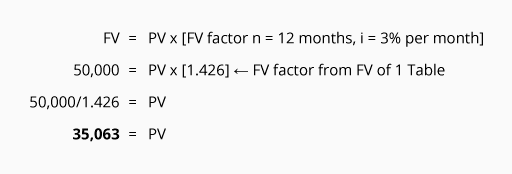The present amount of visitors per day must be 35,063 if a 3% per month compounded increase results in 50,000 visitors per day after 12 months. (You can verify the answer 35,063 by reviewing the table below.)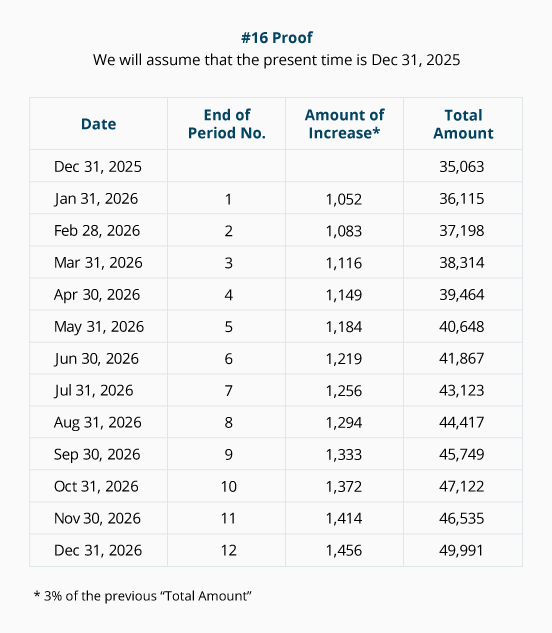If our future value factors were not rounded to only 3 decimal places, the present number of visitors per day at December 31, 2022 would have been 35,069 and that would result in 50,000 at Dec 31, 2023.